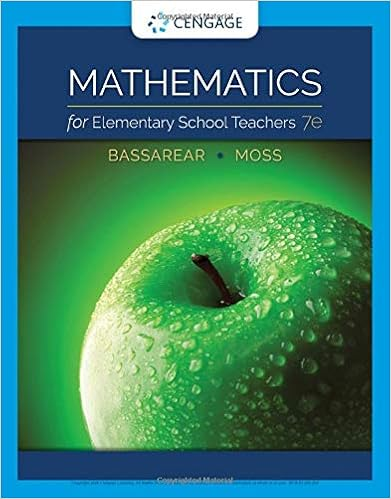N diverges so by the comparison test the series ln n

• Homework Help
• 4
• 87% (15) 13 out of 15 people found this document helpful

This preview shows page 1 - 3 out of 4 pages.

We have textbook solutions for you!
The document you are viewing contains questions related to this textbook.The document you are viewing contains questions related to this textbook.
Chapter 3 / Exercise 23
Mathematics for Elementary School Teachers
BassarearExpert Verified
I I I C C I 3. (1 pt) Consider the series n = 1 1 n ( n + 3 ) Determine whether the series converges, and if it converges, determine its value. Converges (y/n): Value if convergent (blank otherwise): Correct Answers: Y 0.611111111111111 4. (1 pt) Match each of the following with the correct state-
ment. C stands for Convergent, D stands for Divergent. 1. 8 n 2. n = 1 1 2 + 4 n 6 3. n = 2 2 n 8 - 9 4. n = 1 2 n ( n + 3 ) 5. n = 1 8 + 7 n 8 + 2 n n = 1 ln ( n )
1
1 n diverges, so by the Comparison Test, the series ln ( n ) n diverges. 6. For all n > 2, ln ( n ) n 2 > 1 n 2 , and the series 1 n 2 converges, so by the Comparison Test, the series ln ( n ) n 2 converges. 5. (1 pt) Use the ratio test to determine whether n = 14 n + 9 n ! converges or diverges. (a) Find the ratio of successive terms. Write your answer as a fully simplified fraction. For n 14, lim n a n + 1
We have textbook solutions for you!
The document you are viewing contains questions related to this textbook.The document you are viewing contains questions related to this textbook.
Chapter 3 / Exercise 23
Mathematics for Elementary School Teachers
BassarearExpert Verified
(b) Evaluate the limit in the previous part. Enter as infinity and - as -infinity. If the limit does not exist, enter DNE. (c) By the ratio test, does the series converge, diverge, or is the test inconclusive? ? 6. (1 pt) Use the ratio test to determine whether 2 n converges or diverges. (a) Find the ratio of successive terms. Write your answer as a fully simplified fraction. For n 26, lim n (b) Evaluate the limit in the previous part. Enter as infinity and - as -infinity. If the limit does not exist, enter DNE. (c) By the ratio test, does the series converge, diverge, or is the test inconclusive? ?
•••